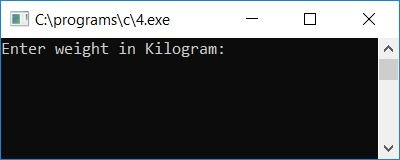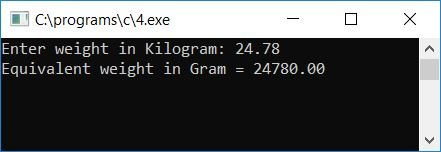# C program to convert kilograms to grams

In this article, we will learn how to create a program in C that will ask the user to enter a weight in kilograms and then convert and print its equivalent weight in grams. But, before we get into the program, let's first understand the difference between a kilogram and a gram.

### The Kilogram and Gram Relationship

The relationship between a kilogram and a gram is:

`1 kilogram = 1000 grams`

Kilogram is abbreviated or represented as kg, whereas gram is represented as g. As a result, the relationship between these two can be written as follows:

`1 kg = 1000 g`

Now let's move on to the program.

## Kilogram to Gram Conversion in C

The question is: write a program in C that converts the given weight (by the user at run-time) from kilograms to grams. The answer to this question is given below:

```#include<stdio.h>
#include<conio.h>
int main()
{
float kg, g;
printf("Enter weight in Kilogram: ");
scanf("%f", &kg);
g = kg*1000;
printf("Equivalent weight in Gram = %0.2f", g);
getch();
return 0;
}```

Because the program was written in the Code::Blocks IDE, here is a snapshot of the sample run after a successful build and run:Supply any input or weight in kilograms (say 5) and press the ENTER key to see the same weight in grams as shown in the second snapshot given here:Let's take another sample run. Enter the weight in kilograms, say 24.78, to see its equivalent weight in grams:Here are some of the main steps used in the above program:

• Receive weight input (in kilograms) from the user at run-time.
• 1 kilogram is equal to 1000 grams.
• To convert 1 kg to gram, multiply the given value (in kilograms) by 1000 to get the same weight value in gram.
• That is, kg * 1000 (kg is the value of weight given by the user in kilograms) gets initialized to any variable, say g (which holds equivalent weight in grams).
• At the end of the program, print the value of g.
• For example, if the user enters their weight in kilograms as 12.43, then kg*1000 or 12.43*1000 or 12430 gets initialized to g.
• Print the value of g, which will be 12430, as output.
• The %0.2f format specifier is used to print the value up to 2 decimal places only.

C Quiz

« Previous Program Next Program »